# Theory of Computation at Columbia

The Theory of Computation group is a part of the Department of Computer Science in the Columbia School of Engineering and Applied Sciences.

We research the fundamental capabilities and limitations of efficient computation. In addition, we use computation as a lens to gain deeper insights into problems from the natural, social, and engineering sciences. Our active research areas include algorithmic game theory, complexity theory, cryptography, the design and analysis of algorithms, interactive computation and communication, theoretical neuroscience, property testing, the role of randomness in computation, sublinear and streaming algorithms, and the theoretical foundations of machine learning.

Our group is highly collaborative, both within Columbia and among peer institutions. We have a weekly Theory Lunch and a bi-weekly Student Seminar. Most graduate students have (at least) two advisors and collaborate with several professors and other students. Some of our faculty are cross-listed with the IEOR department and the Data Science Institute. We interact with the New York theory community at large through NYCAC, NYC Theory Day, NYC Crypto Day, and the Simons Collaboration on Algorithms and Geometry.

Our department and research group are growing, and we’re always looking for new members and collaborators. If you would like to join our group as a graduate student, please apply to the PhD program in Computer Science at Columbia. Please reach out to faculty directly for inquiries about postdoc positions.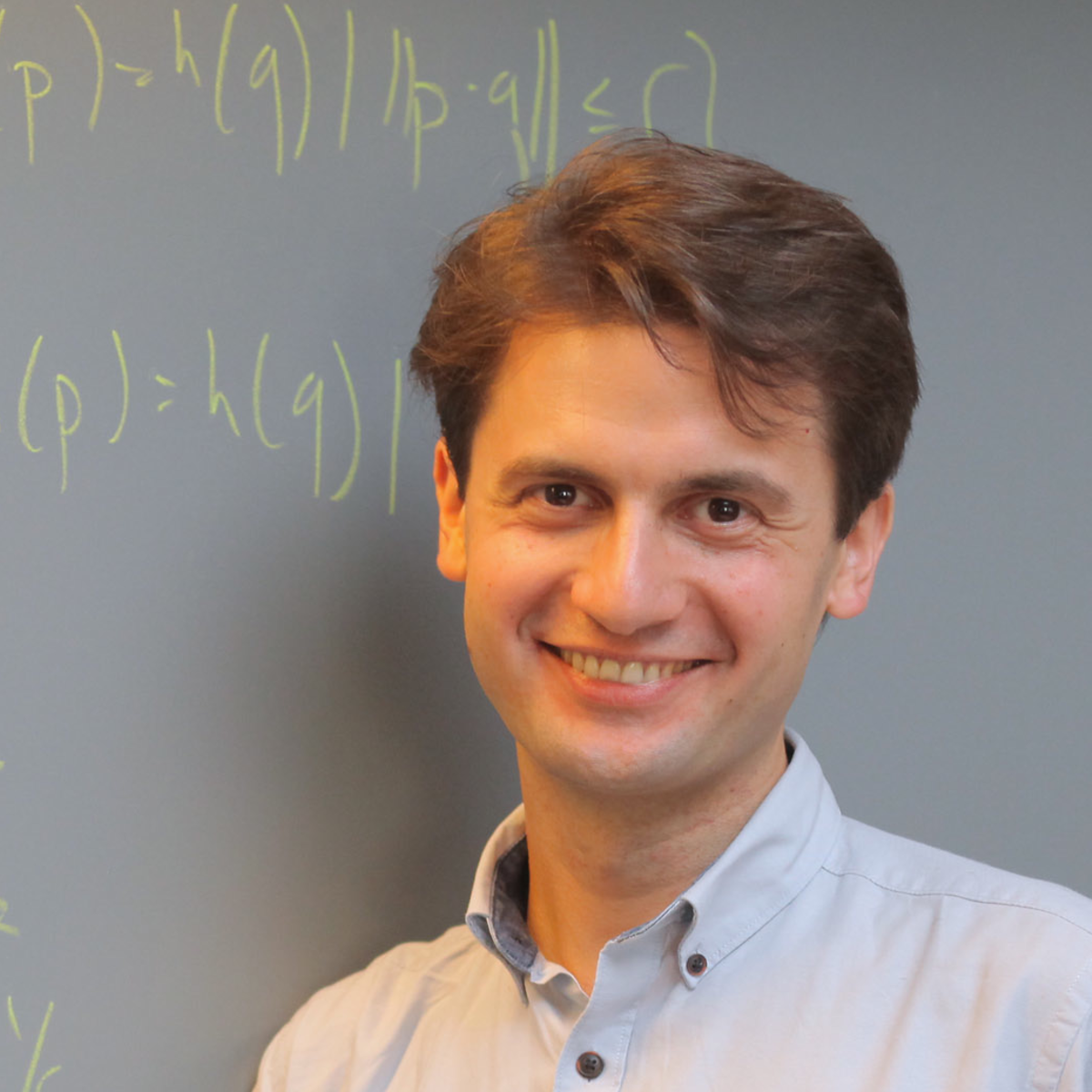Sublinear Algorithms, High-dimensional Geometry, Machine Learning Theory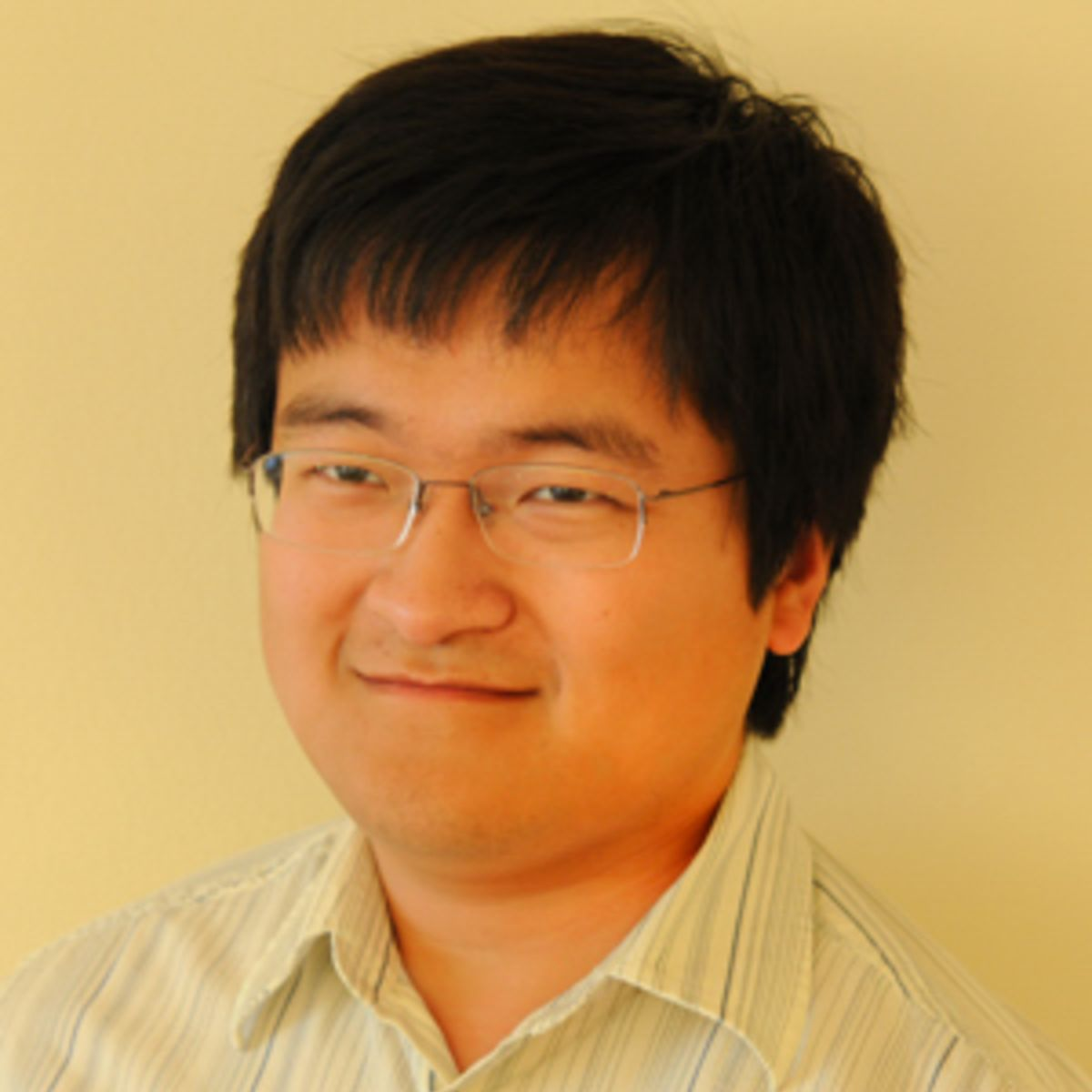Algorithmic Game Theory, Complexity Theory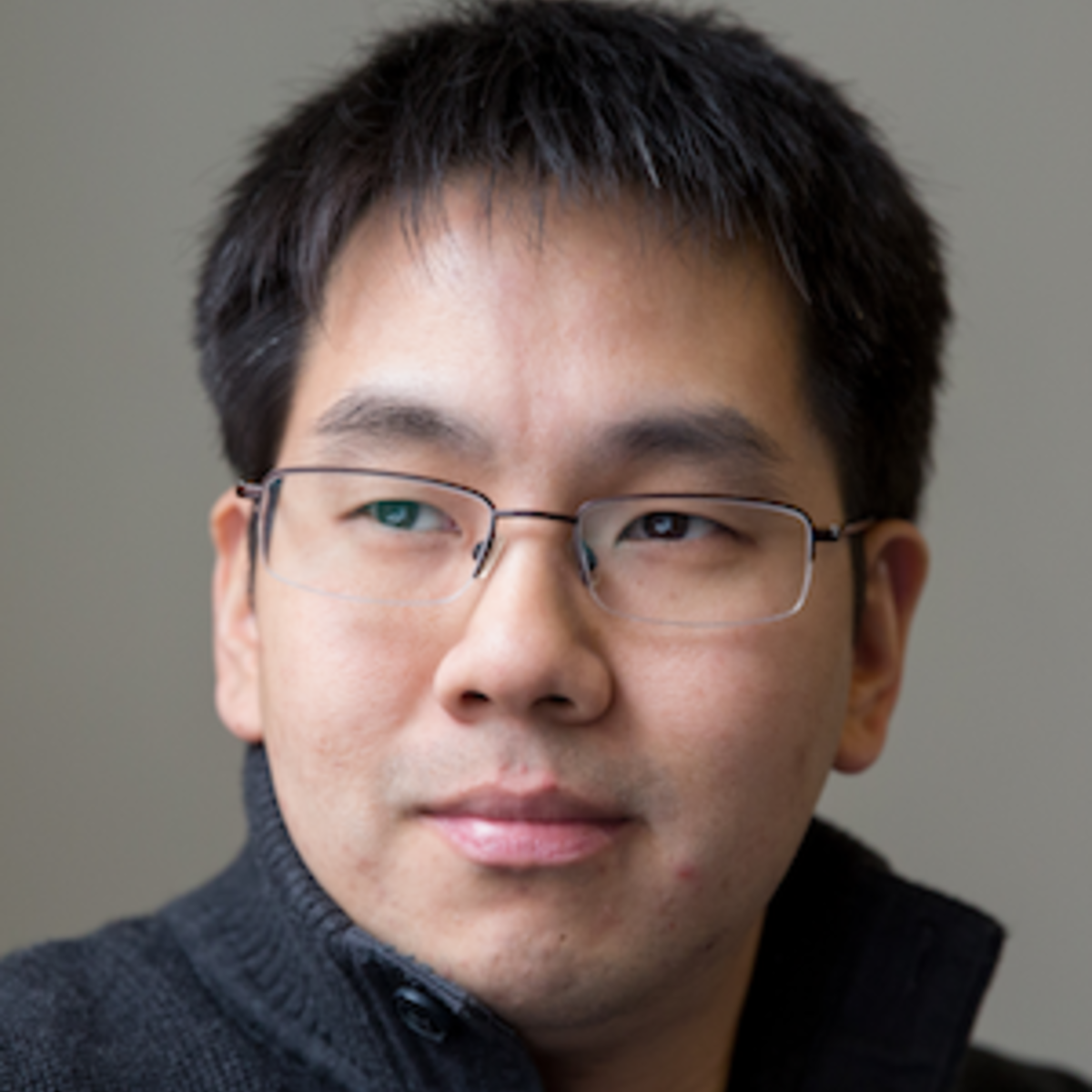Algorithmic Statistics, Machine Learning, Privacy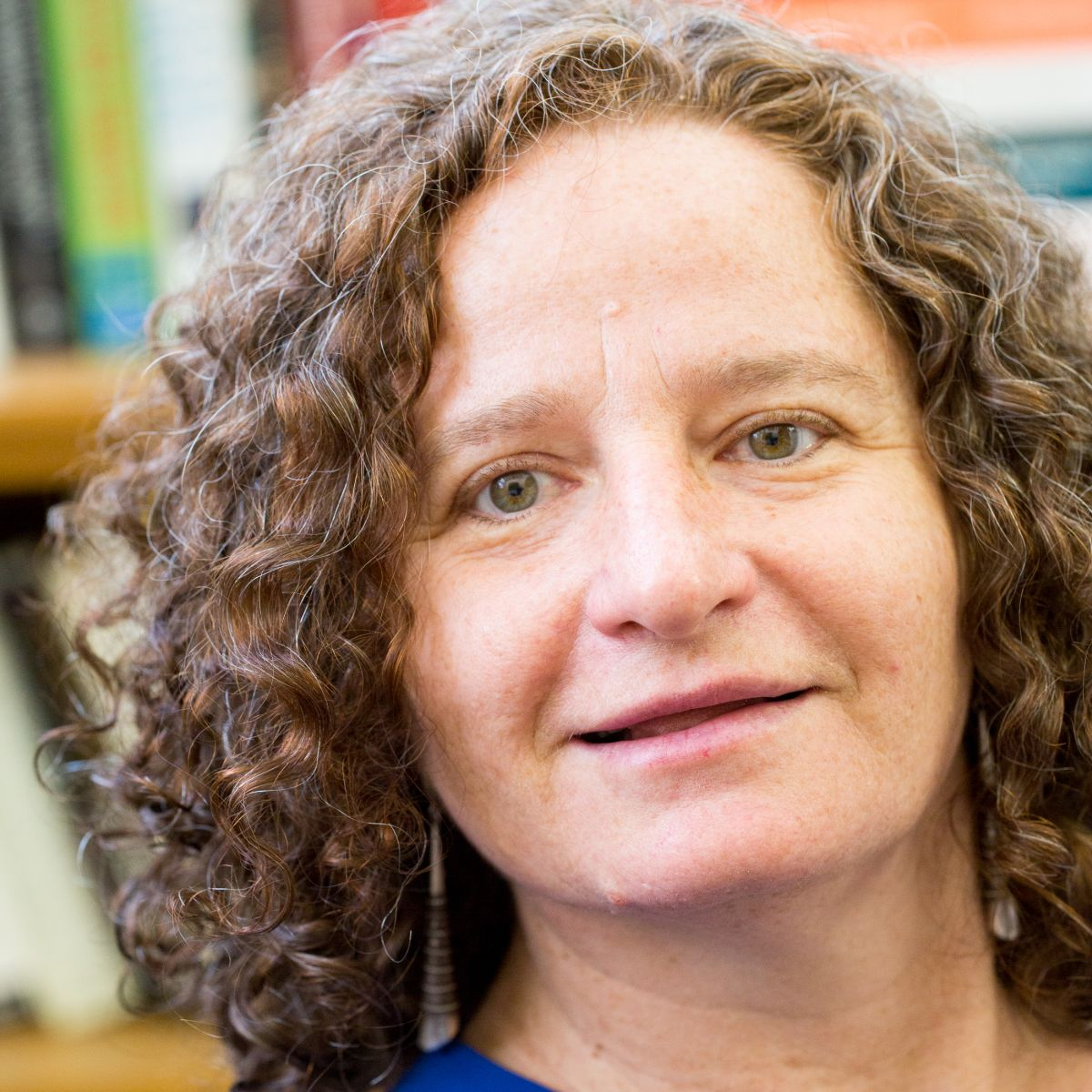Computational Complexity, Cryptography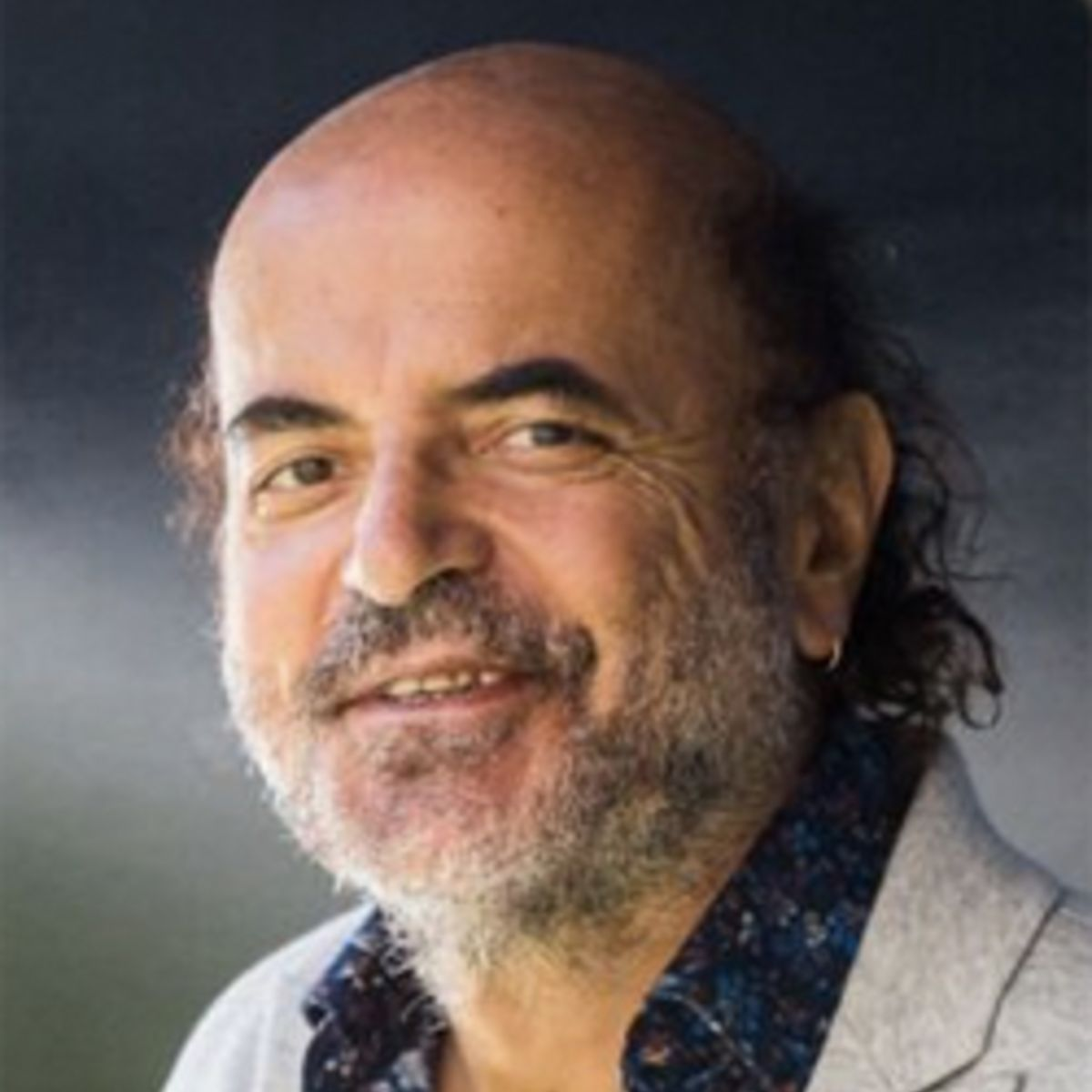Algorithms, Complexity, Algorithmic Game Theory, Evolution, The Brain, Learning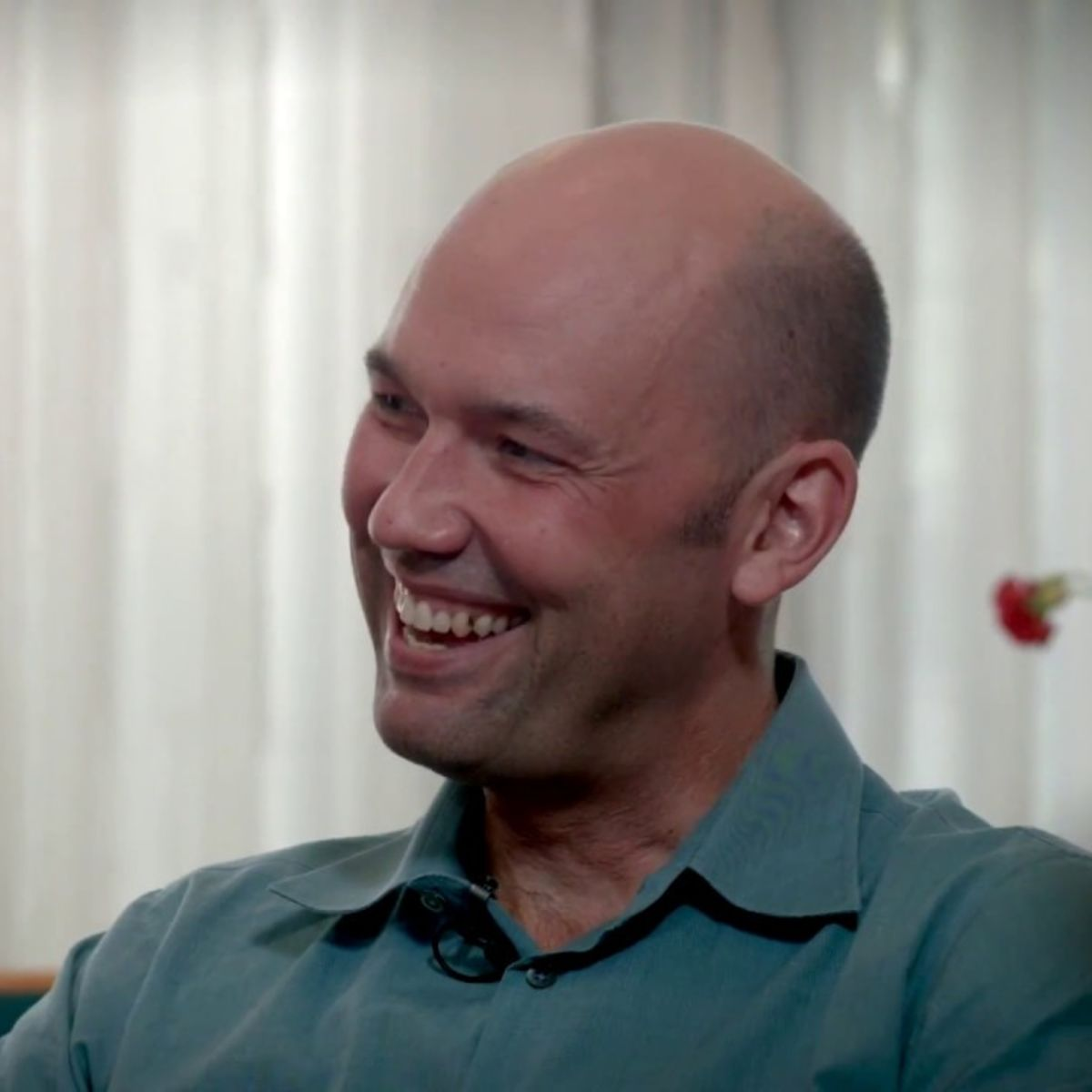Algorithmic Game Theory, Algorithms, Cryptocurrencies, Microeconomics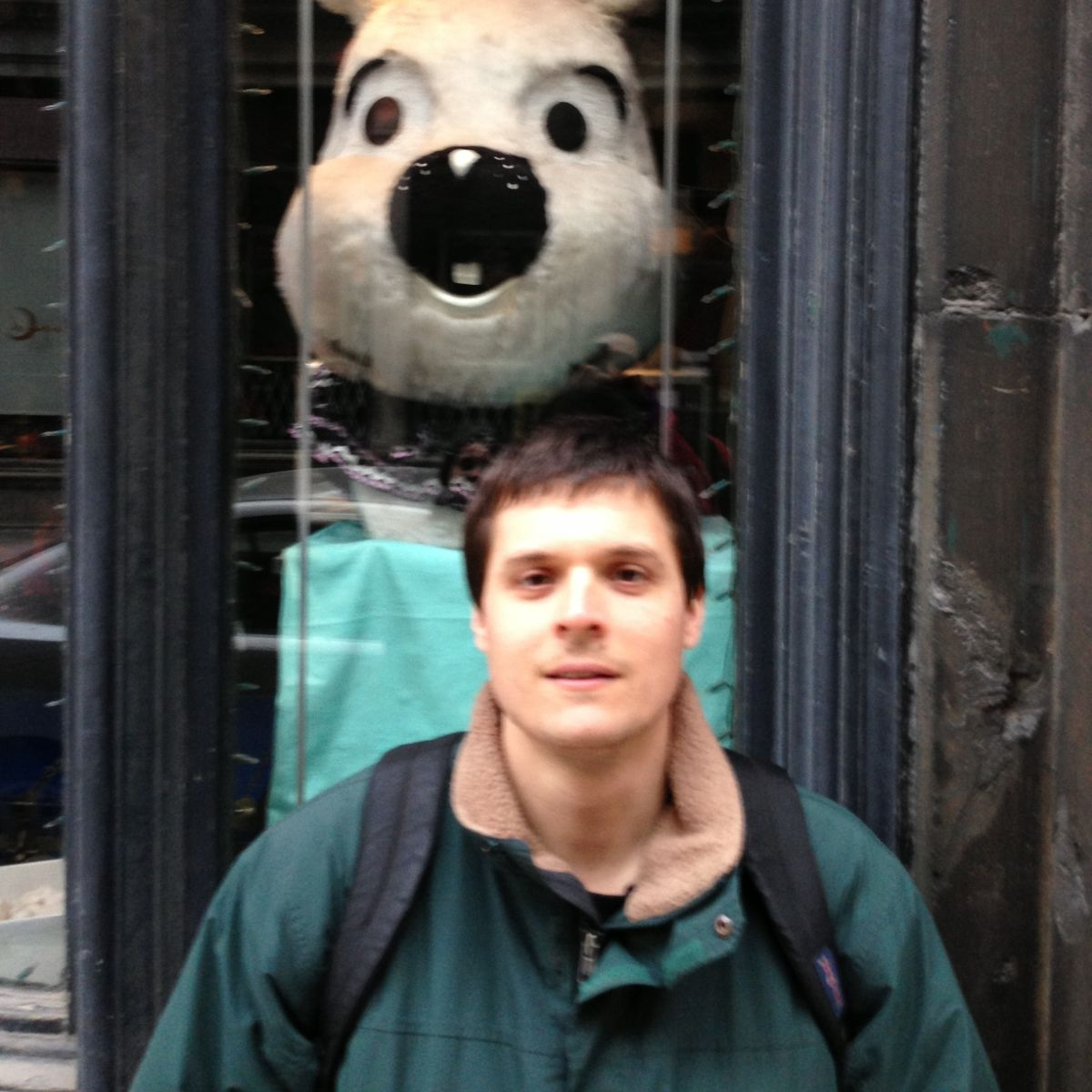Computational Complexity, Learning Theory, Randomness in ComputationAlgorithms, Combinatorial Optimization, Network Algorithms, Scheduling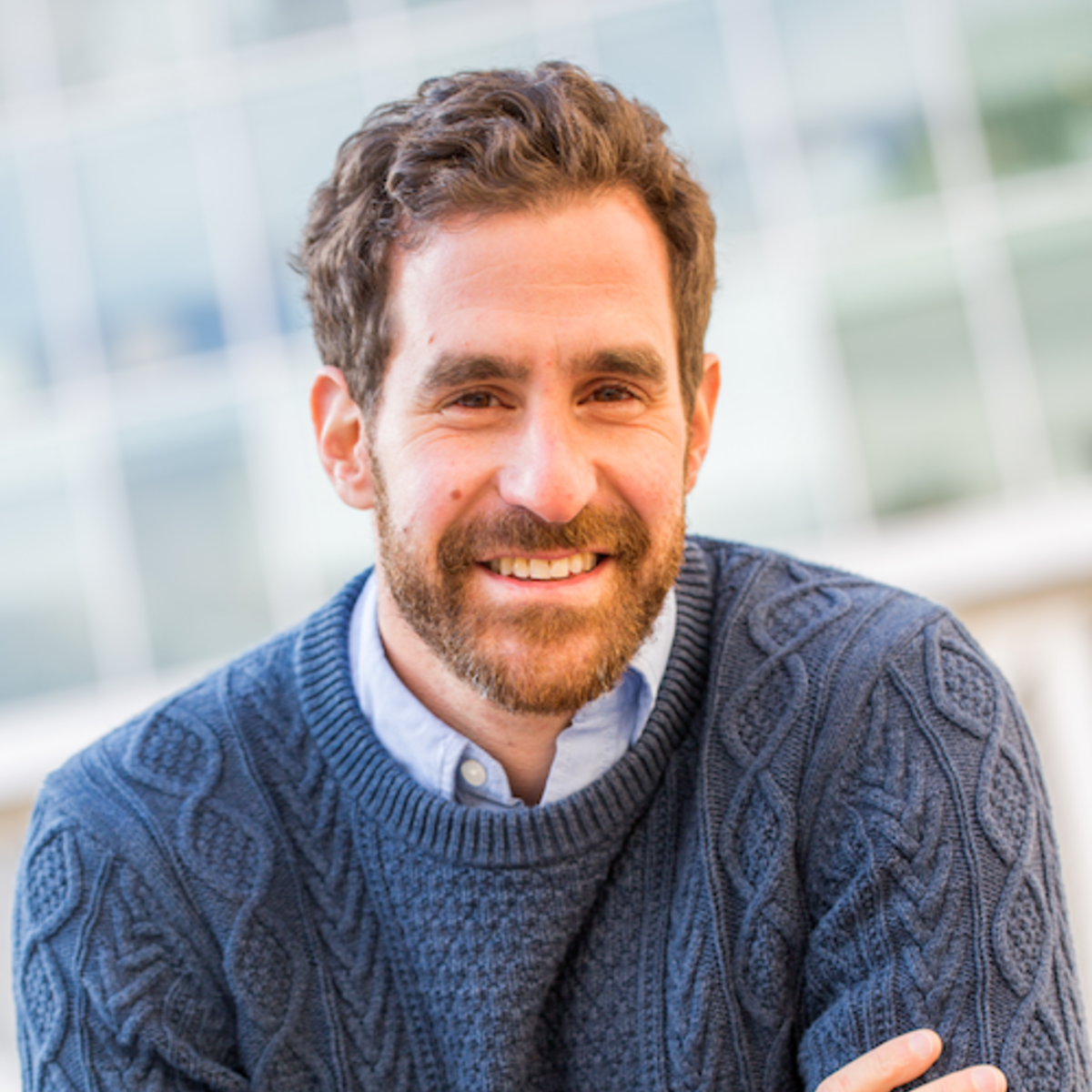Information Theory, Communication Complexity, Data-Structure Lower Bounds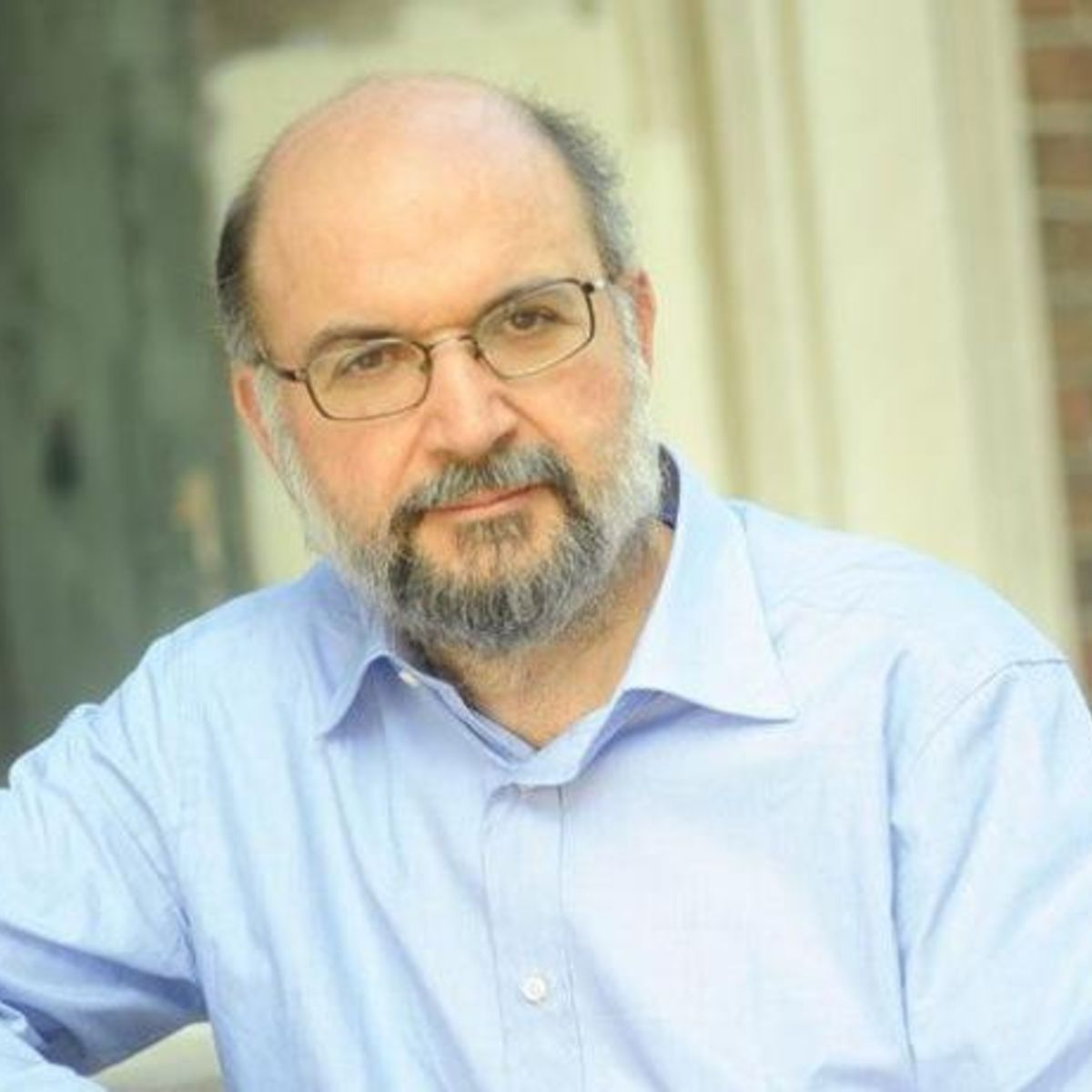Algorithms, Complexity Theory, Combinatorial Optimization, Databases, Testing and Verification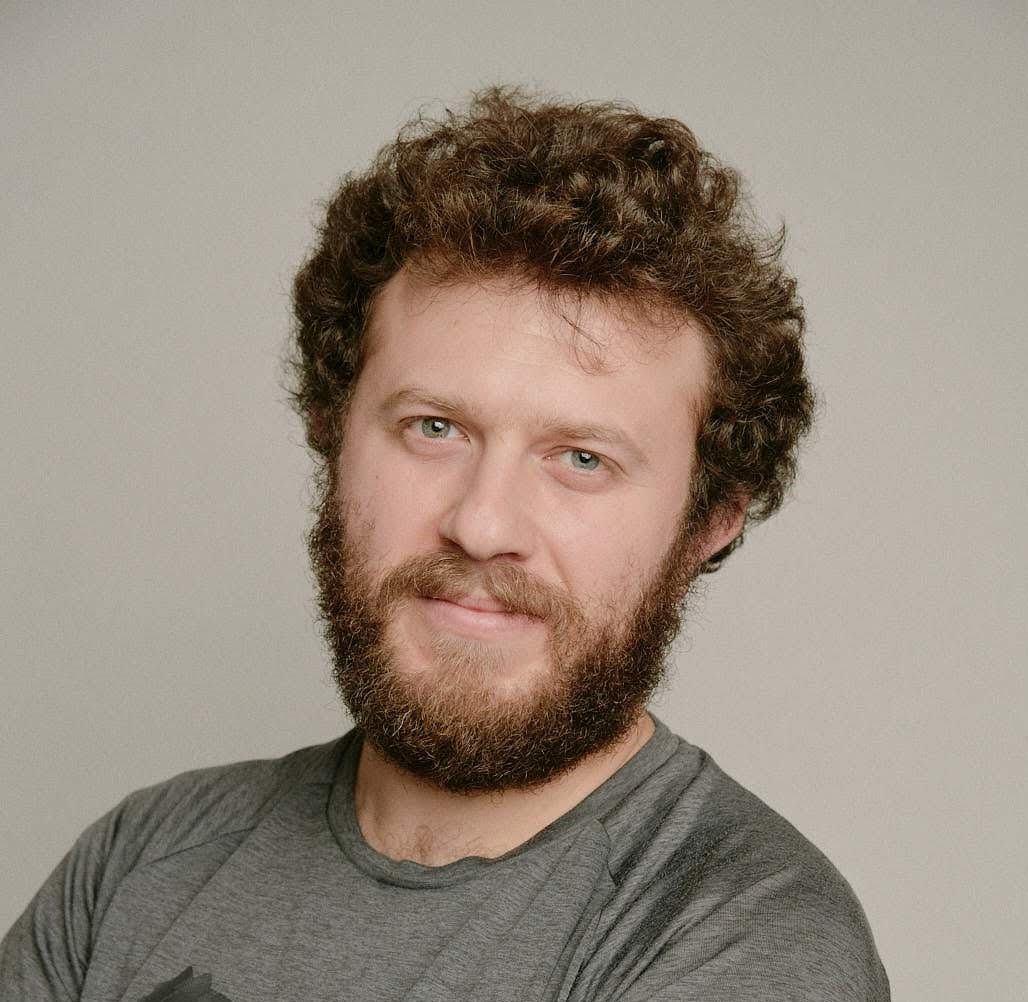Algorithms, Metric Embeddings, ApproximationMechanism Design, Approximation Algorithms, Algorithmic Game TheoryShunhua JiangChengyu LinDan Mitropolsky
Complexity, Cryptography, Theoretical Neuroscience, Language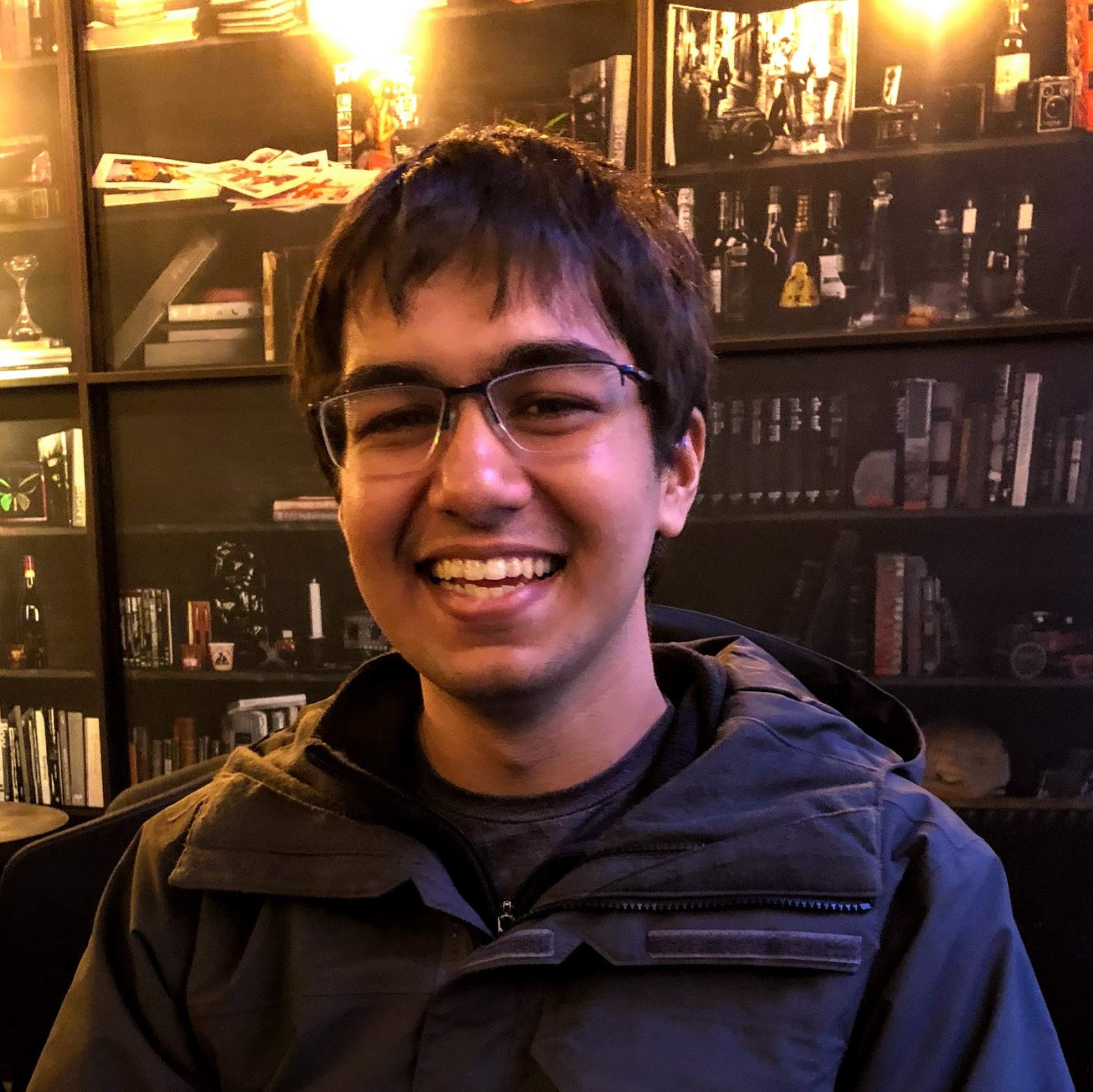Boolean Function Analysis, Complexity Theory, Cryptography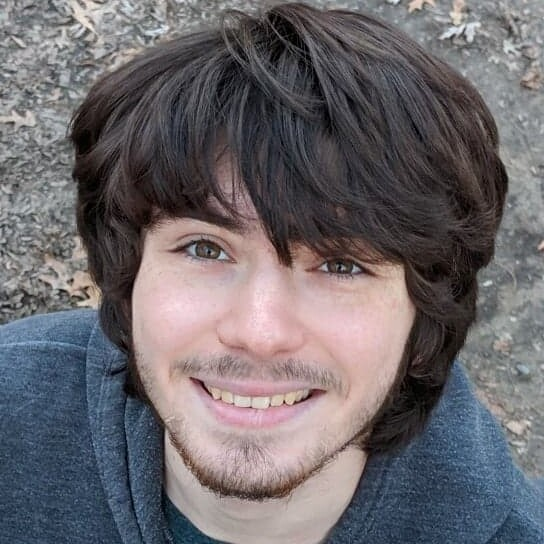Algorithmic Game Theory, Algorithms, ComplexityAlgorithms, Algorithmic Game Theory, Machine Learning, NetworksComplexity Theory, Data Structures, Learning TheoryRandomized Algorithms, Market & Mechanism Design, GraphsMachine Learning Theory, Neural Networks, AI Fairness & AccountabilityNegev Shekel-NosatzkiLearning Theory, Data Structures, Information Theory, Sublinear AlgorithmsAlgorithmic Game Theory, Algorithms, Learning TheoryTheoretical Machine Learning, AlgorithmsAlgorithms, Complexity Theory, Property Testing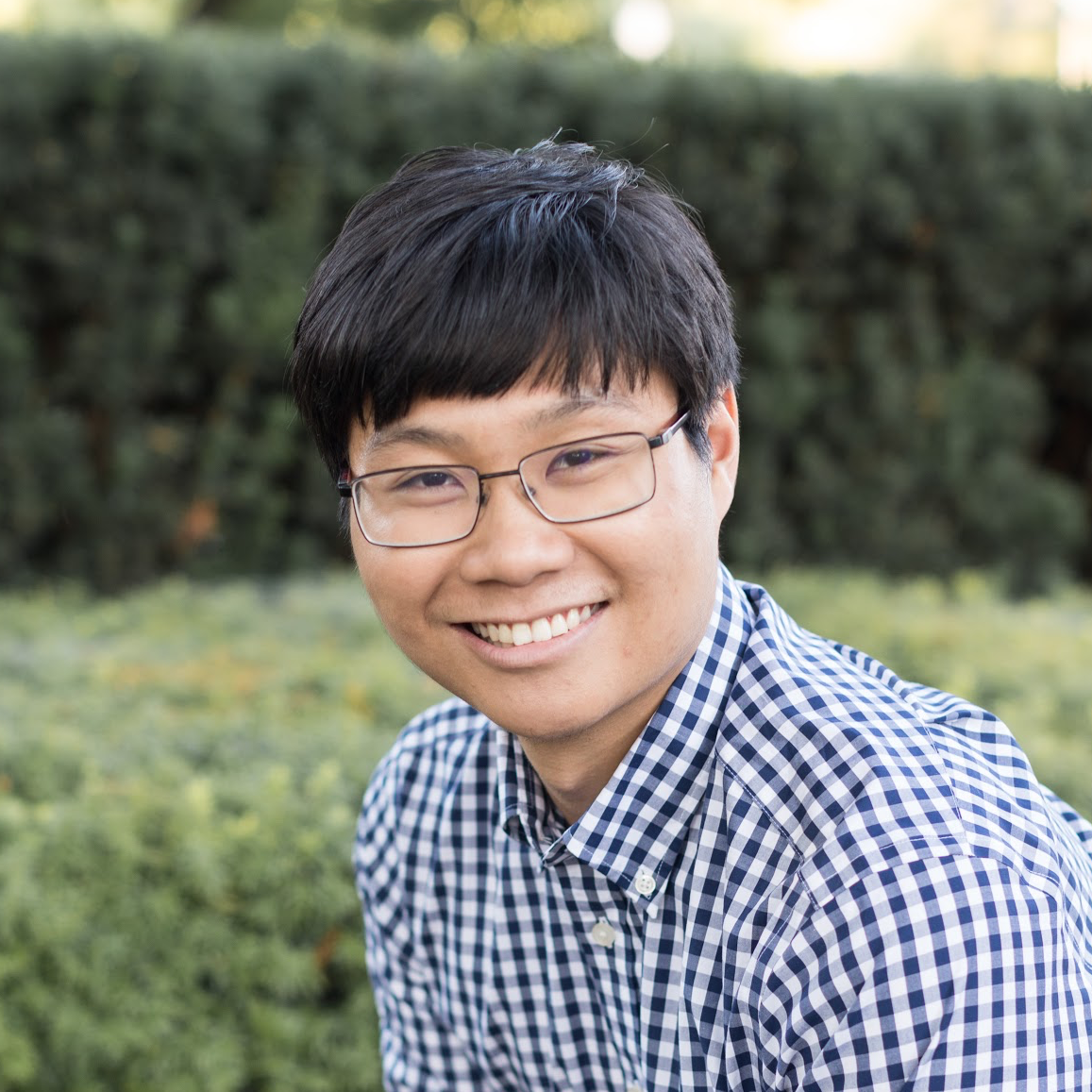Cryptography, Data Structures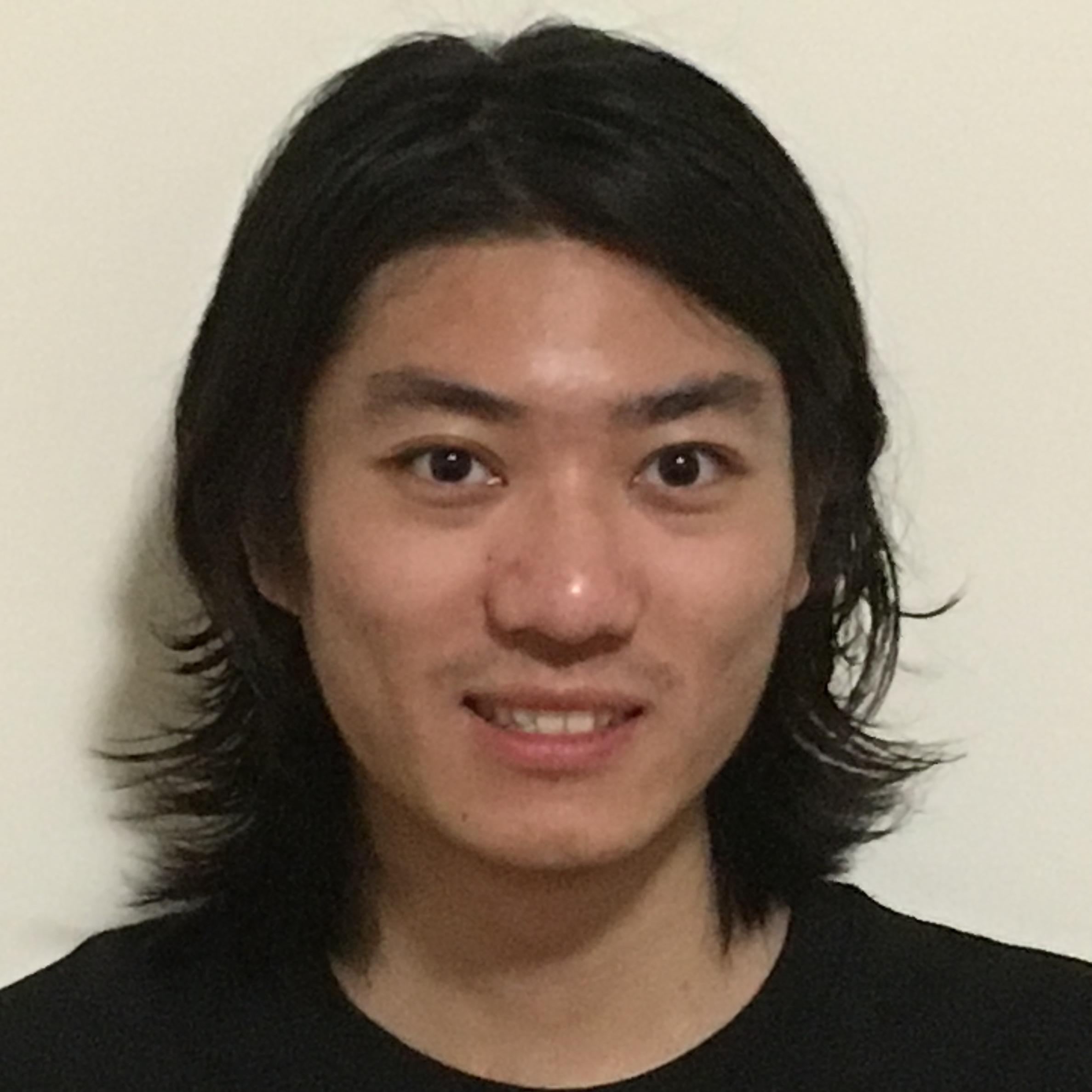Hengjie Zhang
Approximation Algorithms, Data StructuresSublinear Algorithms, High-dimensional Geometry, Machine Learning TheoryAlgorithms, Complexity TheoryComplexity Theory, Property TestingSublinear AlgorithmsCryptography, Information Security, Distributed-Ledger Technology